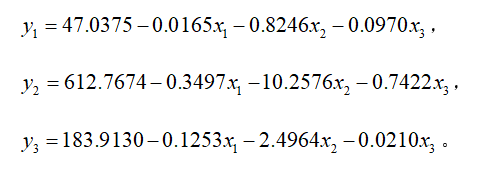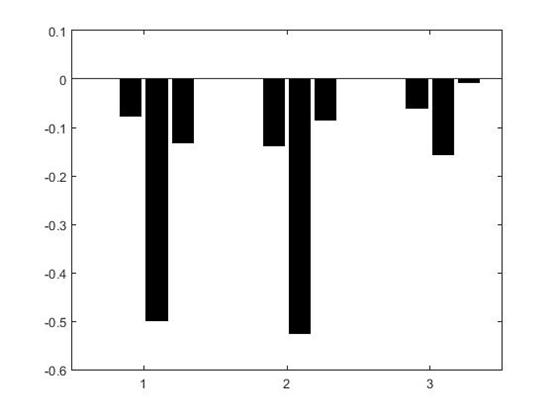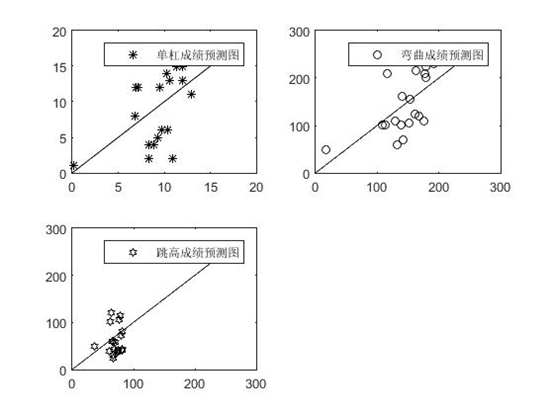• ## 偏回归

千次阅读 2014-07-15 17:03:23
偏相关系数 Partial correlation coefficient 在多元回归分析中，在消除其他...偏回归系数 partial regression coefficient 在多元回归分析中，随机因变量对各个自变量的回归系数，表示各自变量对随机变量的影响程度
偏相关系数
Partial correlation coefficient 在多元回归分析中，在消除其他变量影响的条件下，所计算的某两变量之间的相关系数。偏回归系数partial regression coefficient 在多元回归分析中，随机因变量对各个自变量的回归系数，表示各自变量对随机变量的影响程度

展开全文• 在实际问题中，要研究两组多重相关变量间的相互依赖关系时，可使用的方法有：经典多元线性回归分析（MLR）、主成分回归分析（PCR）、最小二乘回归分析（PLS）。 当两组变量的个数很多，且都存在多重相关性，而观测...
在实际问题中，要研究两组多重相关变量间的相互依赖关系时，可使用的方法有：经典多元线性回归分析（MLR）、主成分回归分析（PCR）、偏最小二乘回归分析（PLS）。
当两组变量的个数很多，且都存在多重相关性，而观测的样本数又较少时，用PLS建立模型具有MLR等方法所没有的优点。
PLS在建模的过程中集中了主成分分析、典型相关分析、线性回归分析方法的特点，除了能提供一个合理的回归模型外，还可以提供一些更深入的信息。
1. 偏最小二乘回归分析概述
假定p个因变量$y_1,\cdots,y_p$与m个自变量$x_1,\cdots,x_m$均为标准化变量。自变量组合因变量组的标准化观测数据矩阵分别为$\bm{A}^{n\times m},\bm{B}^{n\times p}$，偏最小二乘回归分析建模的具体步骤如下：

分别提取两变量组的第一对成分$u_1,v_1$，代表自变量和因变量的线性组合$u_1=\bm{\rho^{(1)T}X}\ ,v_1=\bm{\gamma^{(1)T}Y}$为了回归分析的需要，要求：① $u_1$和$v_1$各自尽可能多地提取所在变量组的变异信息；② $u_1$和$v_1$的相关程度达到最大。
由两组变量集的标准化观测数据矩阵$\bm{A}$和$\bm{B}$，可以计算第一对成分的得分向量，记为$\bm{\hat{u}_1}$和$\bm{\hat{v}_1}$$\bm{\hat{u}_1=A\rho^{(1)}}\ ,\bm{\hat{v}_1=B\gamma^{(1)}}$此时可将以上两个要求化为数学上的条件极值问题$\max(\bm{\hat{u}_1\cdot\hat{v}_1})=\bm{\rho^{(1)T}A^TB\gamma^{(1)}}\\ \text{s.t.}\begin{cases}\bm{\rho^{(1)T}\rho^{(1)}}=||\bm{\rho^{(1)}}||^2=1\\\bm{\gamma^{(1)T}\gamma^{(1)}}=||\bm{\gamma^{(1)}}||^2=1\end{cases}$利用拉格朗日乘数法，将问题转为计算矩阵$\bm{M=A^TBB^TA}$的特征值和特征向量，其中最大特征值对应的单位特征向量就是$\bm{\rho^{(1)}}$，且有$\bm{\gamma^{(1)}}=\cfrac{1}{\lambda_1}\bm{B^TA\rho^{(1)}}$。
建立$y_1,\cdots,y_p$对$u_1$的回归，及$x_1,\cdots,x_m$对$u_1$的回归。假定回归模型为$\begin{cases}\bm{A=\hat{u}_1\sigma^{(1)T}+A_1}\\\bm{B=\hat{u}_1\tau^{(1)T}+B_1}\end{cases}$式中$\bm{\sigma^{(1)}}=[\sigma_{11},\cdots,\sigma_{1m}]^T\ ,\bm{\tau^{(1)}}=[\tau_{11},\cdots,\tau_{1p}]^T$分别为多对一的回归模型中的参数向量；$\bm{A_1,B_1}$是残差阵。则回归系数向量$\bm{\sigma^{(1)}},\bm{\tau^{(1)}}$的最小二乘估计为$\begin{cases}\bm{\sigma^{(1)}=A^T\hat{u}_1/||\hat{u}_1||^2}\\\bm{\tau^{(1)}=B^T\hat{u}_1/||\hat{u}_1||^2}\end{cases}$称$\bm{\sigma^{(1)},\tau^{(1)}}$为模型效应负荷量。
若残差阵$\bm{B_1}$中元素的绝对值近似为0，则认为用第一个成分建立的回归式精度已经满足需要了，可以停止抽取成分。否则用残差阵$\bm{A_1,B_1}$代替$\bm{A,B}$，重复以上步骤。得$\begin{cases}\bm{A=\hat{u}_1\sigma^{(1)T}+\hat{u}_2\sigma^{(2)T}+A_2}\\\bm{B=\hat{u}_1\tau^{(1)T}+\hat{u}_2\tau^{(2)T}+B_2}\end{cases}$
设$n\times m$数据阵$\bm{A}$的秩为$r\leqslant\min(n-1,m)$，则存在r个成分$u_1,\cdots,u_r$，使得$\begin{cases}\bm{A=\hat{u}_1\sigma^{(1)T}+\cdots+\hat{u}_r\sigma^{(r)T}+A_r}\\\bm{B=\hat{u}_1\tau^{(1)T}+\cdots+\hat{u}_r\tau^{(r)T}+B_r}\end{cases}$将$u\sim x$带入$y\sim u$，即得$y\sim x$的偏最小二乘回归方程。
交叉有效性检验：一般情况下，偏最小二乘法并不需要选用存在的r个成分$u_1,\cdots,u_r$来建立回归式，只选用前$l$个成分即可得到预测能力较好地回归模型。对于建模所需提取的成分个数$l$，可通过交叉有效性检验来确定。
每次舍去第$i(i=1,2,\cdots,n)$个观测数据，对余下n-1个观测数据用偏最小二乘回归方法建模，并考虑抽取$h(h\leqslant r)$个成分后拟合的回归式，然后把舍去的自变量组第i个观测数据代入所拟合的回归方程式，得到$y_j(j=1,2,\cdots,p)$在第i个观测点上的预测值$\hat{b}_{(i)j}(h)$。
对$i=1,2,\cdots,n$重复以上的验证，抽取h个成分时第j个因变量$y_j$的预测误差平方和为$\operatorname{PRESS}_j(h)=\sum_{i=1}^n[b_{ij}-\hat{b}_{(i)j}(h)]^2$$\bm{Y}=[y_1,\cdots,y_p]^T$的预测误差平方和为$\operatorname{PRESS}(h)=\sum_{j=1}^p\operatorname{PRESS}_j(h)$另外，再采用所有的样本点，拟合含h个成分的回归方程。此时，记第i个样本点的预测值为$\hat{b}_{ij}(h)$，则可以定义$y_j$的误差平方和为$\operatorname{SS}_j(h)=\sum_{i=1}^n[b_{ij}-\hat{b}_{ij}(h)]^2$定义$\bm{Y}$的误差平方和为$\operatorname{SS}(h)=\sum_{j=1}^p\operatorname{SS}_j(h)$当$\operatorname{PRESS}(h)$达到最小值时，对应的h即为所求得成分个数l。定义交叉有效性为$Q^2_h=1-\cfrac{\operatorname{PRESS}(h)}{\operatorname{SS}(h-1)}$在建模的每一步计算结束前，均进行交叉有效性检验，如果将限制值设为0.05，在第h步有$Q_h^2<1-0.95^2=0.0975$，则模型达到精度要求，可停止提取成分；否则表示第h步提取的$u_h$成分边际贡献显著，应继续第h+1步计算。

2. Matlab偏最小二乘回归命令plsregress
[XL,YL,XS,YS,BETA,PCTVAR,MSE,stats] = plsregress(X,Y,ncomp)


X为[n,m]的自变量数据阵；Y为[n,p]的因变量数据阵；ncomp为成分个数，默认为min(n-1,m)
XL为[m,ncomp]的负荷量矩阵$\bm{\sigma}$；YL为[p,ncomp]的负荷量矩阵$\bm{\tau}$；XS为$\bm{\hat{u}}$的得分矩阵；YS为$\bm{\hat{v}}$的得分矩阵
BETA的每一列对应$y\sim x$的回归表达式；PCTVAR是一个两行的矩阵，两行分别代表每个元素对应自变量和因变量提出成分的贡献率；MSE是一个两行的矩阵，两行分别代表自变量和因变量对应提出成分之间回归方程的剩余标准差
stats返回4个值，其中stats.W的每一列对应特征向量$\bm{\rho}$，Matlab算得的特征向量不是单位向量


展开全文数学建模
• 最小二乘回归分析实例plsregress命令
偏最小二乘回归分析实例

原文：http://blog.csdn.net/qq_34861102/article/details/77102683

plsregress命令

步骤 数据标准化
求相关系数矩阵
分别提出自变量组合因变量组的成分
求两个成分对标准化指标变量和成分变量之间的回归方程
求因变量组与自变量组之间的回归方程
模型的解释与检验
实例代码

ab0 = load('data.txt');
%均值和方差
mu = mean(ab0);
sig = std(ab0);
%标准化以后的自变量和因变量数据
a = ab(:,[1:end-1]);
b = ab(:,end);
[XL YL XS YS BETA PCTVAR MSE status] = plsregress(a,b);
%求自变量的主成分系数
xw = a\XS;
%求因变量的主成分系数
yw = b\YS;
ncomp = input('请根据PCTVAR的值确定提出成分对的个数');

[XL2 YL2 XS2 YS2 BETA2 PCTVAR2 MSE2 status2] = plsregress(a,b,ncomp);
%自变量的个数
n = size(a,2);
%原始数据回归方程的常数项
beta3(1) = mu(end) - mu(1:n)./sig(1:n)*BETA2([2:end]).*sig(end);
beta3([2:n+1]) = (1./sig(1:n))'*sig(n+1:end).*BETA2([2:end]);
%直方图
bar(BETA2','k');
%y的预测值
yhat = repmat(beta3(1),[size(a,1),1]) + ab0(:,[1:n])*beta3([2:end])';
%预测值的最大值
ymax = max([yhat;ab0(:,end)]);

展开全文实例
• 小二乘回归提供一种多对多线性回归建模的方法，特别当两组变量的个数很多，且都存在多重相关性，而观测数据的数量（样本量）又较少时，用小二乘回归建立的模型具有传统的经典回归分析等方法所没有的优点。...
给大家安利一款朋友开发的自研国产数据分析基础工具，一键式自动分析，自动生成分析模板，5分钟掌握主流61个统计类数学模型（几乎涵盖SPSS绝大部分功能），以及23个有监督机器学习（包括随机森林，SVM，XGBoost等）

PS：巨方便简单上手，貌似现在是免费

官网：www.mpaidata.com   mpai数据科学平台

偏小二乘回归提供一种多对多线性回归建模的方法，特别当两组变量的个数很多，且都存在多重相关性，而观测数据的数量（样本量）又较少时，用偏小二乘回归建立的模型具有传统的经典回归分析等方法所没有的优点。

偏小二乘回归分析在建模过程中集中了主成分分析，典型相关分析和线性回归分析方法的特点，因此在分析结果中，除了可以提供一个更为合理的回归模型外，还可以同时完成一些类似于主成分分析和典型相关分析的研究内容，提供更丰富、深入的一些信息。

例 本节采用兰纳胡德（Linnerud）给出的关于体能训练的数据进行偏小二乘回归建模。在这个数据系统中被测的样本点，是某健身俱乐部的20位中年男子。被测变量分为两组。第一组是身体特征指标X，包括：体重、腰围、脉搏。第二组变量是训练结果指标Y，包括：单杠、弯曲、跳高。原始数据见表1。

表2给出了这6个变量的简单相关系数矩阵。从相关系数矩阵可以看出，体重与腰围是正相关的；体重、腰围与脉搏负相关；而在单杠、弯曲与跳高之间是正相关的。从两组变量间的关系看，单杠、弯曲和跳高的训练成绩与体重、腰围负相关，与脉搏正相关。

表 1  体能训练数据

No

体重( )

腰围( )

脉搏( )

单杠( )

弯曲( )

跳高( )

1

191

36

50

5

162

60

2

189

37

52

2

110

60

3

193

38

58

12

101

101

4

162

35

62

12

105

37

5

189

35

46

13

155

58

6

182

36

56

4

101

42

7

211

38

56

8

101

38

8

167

34

60

6

125

40

9

176

31

74

15

200

40

10

154

33

56

17

251

250

11

169

34

50

17

120

38

12

166

33

52

13

210

115

13

154

34

64

14

215

105

14

247

46

50

1

50

50

15

193

36

46

6

70

31

16

202

37

62

12

210

120

17

176

37

54

4

60

25

18

157

32

52

11

230

80

19

156

33

54

15

225

73

20

138

33

68

2

110

43

均值

标准差

178.6

24.6905

35.4

3.202

56.1

7.2104

9.45

5.2863

145.55

62.5666

70.3

51.2775

表 2 相关系数矩阵

1

0.8702

-0.3658

-0.3897

-0.4931

-0.2263

0.8702

1

-0.3529

-0.5522

-0.6456

-0.1915

-0.3658

-0.3529

1

0.1506

0.225

0.0349

-0.3897

-0.5522

0.1506

1

0.6957

0.4958

-0.4931

-0.6456

0.225

0.6957

1

0.6692

-0.2263

-0.1915

0.0349

0.4958

0.6692

1

MATLAB源代码：

clc,clear
mu=mean(ab0);sig=std(ab0); %求均值和标准差
rr=corrcoef(ab0);   %求相关系数矩阵
ab=zscore(ab0); %数据标准化
a=ab(:,[1:3]);b=ab(:,[4:end]);  %提出标准化后的自变量和因变量数据
[XL,YL,XS,YS,BETA,PCTVAR,MSE,stats] =plsregress(a,b);
xw=a\XS;  %求自变量提出成分系数，每列对应一个成分，这里xw等于stats.W
yw=b\YS;  %求因变量提出成分的系数
a_0=PCTVAR(1,:);b_0=PCTVAR(2,:);
a_1=cumsum(a_0);b_1=cumsum(b_0);
i=1;%赋初始值
%判断提出成分对的个数
while ((a_1(i)<0.9)&(a_0(i)>0.05)&(b_1(i)<0.9)&(b_0(i)>0.05))
i=i+1;
end
ncomp=i;
fprintf('%d对成分分别为：\n',ncomp);
for i=1:ncomp
fprintf('第%d对成分：\n',i);
fprintf('u%d=',i);
for k=1:3%此处为变量x的个数
fprintf('+(%f*x_%d)',xw(k,i),k);
end
fprintf('\n');
fprintf('v%d=',i);
for k=1:3%此处为变量y的个数
fprintf('+(%f*y_%d)',yw(k,i),k);
end
fprintf('\n');
end
[XL2,YL2,XS2,YS2,BETA2,PCTVAR2,MSE2,stats2] =plsregress(a,b,ncomp);
n=size(a,2); m=size(b,2);%n是自变量的个数,m是因变量的个数
beta3(1,:)=mu(n+1:end)-mu(1:n)./sig(1:n)*BETA2([2:end],:).*sig(n+1:end); %原始数据回归方程的常数项
beta3([2:n+1],:)=(1./sig(1:n))'*sig(n+1:end).*BETA2([2:end],:); %计算原始变量x1,...,xn的系数，每一列是一个回归方程
fprintf('最后得出如下回归方程：\n')
for i=1:3%此处为变量y的个数
fprintf('y%d=%f',i,beta3(1,i));
for j=1:3%此处为变量x的个数
fprintf('+(%f*x%d)',beta3(j+1,i),j);
end
fprintf('\n');
end
bar(BETA2','k')   %画直方图
fprintf('因变量y的预测值：\n');
yhat=repmat(beta3(1,:),[size(a,1),1])+ab0(:,[1:n])*beta3([2:end],:);  %求y1,..,ym的预测值
disp(yhat);
ymax=max([yhat;ab0(:,[n+1:end])]); %求预测值和观测值的最大值
%下面画y1,y2,y3的预测图，并画直线y=x
figure, subplot(2,2,1)
plot(yhat(:,1),ab0(:,n+1),'*',[0:ymax(1)],[0:ymax(1)],'Color','k')
legend('单杠成绩预测图',2)
subplot(2,2,2)
plot(yhat(:,2),ab0(:,n+2),'O',[0:ymax(2)],[0:ymax(2)],'Color','k')
legend('弯曲成绩预测图',2)
subplot(2,2,3)
plot(yhat(:,3),ab0(:,end),'H',[0:ymax(3)],[0:ymax(3)],'Color','k')
legend('跳高成绩预测图',2)


该MATLAB源代码结果分析：根据PCTVAR可知，前两个成分解释自变量的比率为92.13%，只需要取两对成分即可。故ncomp的值应为2，beta3为最后求解出的回归方程。图1 回归系数的直方图展开全文• 1.回归分析概念：回归分析就利用样本（已知数据），产生拟合方程，从而（对未知数据）进行预测。 2.用途：预测，判别合理性。 3.分类：线性回归分析（一元线性、多元线性、广义线性），非线性（逻辑回归） 4.说明：...
• 当两组变量的个数很多，且都存在多重相关性，而观测数据的数量（样本量）又较少时，用最小二乘回归建立模型 11.1 分析概述 在自变量集中提出第一成分u（尽可能多的提取原自变量中的变异信息），同时在因变量集中...数学建模
• 最小二乘回归(PLS-Partial Least Squares)是一种新型的多元统计数据分析方法，是一种多因变量对多自变量的回归建模方法，是对最小二乘方法的推广。 优点： 1）提供了一种多因变量对多自变量的回归建模方法； 2）...机器学习 深度学习 数据挖掘 数学建模
• ## 回归分析算法

千次阅读 2018-10-29 21:21:07
回归”名词的由来 1889年，英国著名统计学家Francils Galton在研究父代与子代身高之间的关系时发现：身材较高的父母，他们的孩子也较高，但这些孩子的平均身高并没有他们的父母的平均身高高；身材较矮的父母，他们...机器学习
• ## 偏最小二乘（pls）回归分析 matlab

万次阅读 多人点赞 2018-09-08 19:41:24
最小二乘回归特别适合当预测矩阵比观测的有更多变量，以及X的值中有多重共线性的时候。通过投影预测变量和观测变量到一个新空间来寻找一个线性回归模型。 一、数学原理 为了实现最小二乘回归的基本思想，要求...
• 数值型自变量和数值型因变量之间关系的分析方法--相关与回归分析 相关与回归是处理变量之间关系的一种统计方法。 （1）从所处理的变量多少来看 若研究的是两个变量之前的关系，则称为简单相关与简单回归分析； ...相关关系
• ## 多元相关分析与多元回归分析

万次阅读 多人点赞 2018-10-27 17:13:02
什么是回归分析 分析步骤 回归分析与相关分析的主要区别 一元线性相关分析 一元线性回归分析 建模 方差分析检验  t检验 多元回归分析模型建立 线性回归模型基本假设 多元回归分析用途 多元线性相关分析 ...
• 密西根大学的弗耐尔教授称最小二乘回归为第二代回归分析方法。 最小二乘回归方法在统计应用中的重要性主要的有以下几个方面： （1）最小二乘回归是一种多因变量对多自变量的回归建模方法。 （2）最小二乘...
• 0.最小二乘回归集成了多元线性回归、主成分分析和典型相关分析的优点，在建模中是一个更好的选择，并且MATLAB提供了完整的实现，应用时主要的问题是： 注意检验，各种检验参数：有关回归的检验以及有关多元分析...
• ## sklearn 回归分析

千次阅读 2018-06-04 19:31:27
机器学习的基本问题1.有监督学习：通过已知的输入和输出，寻找...1)聚类问题2)成分分析：降低维度，从多个特征中筛选部分最重要的特征1.线性回归介绍略，线性回归大家学过一点统计学的应该都知道吧可能要导入的包imp...python 机器学习
• Reference: 李卫东，2008，《应用多元统计分析》 回归分析 英文名称：regression analysis 定义：研究一个随机变量Y对另一个(X)或一组(X1，X2，…，Xk)变量的相依关系的统计分析方法。...reference
• 摘要 一元线性回归可以说是数据分析中非常简单的一个知识点，有一点点统计、分析、建模经验的人都知道这个分析的含义，也会用各种工具来做这个分析...在回归分析中，只包括一个自变量和一个因变量，且二者的关系可...
• SPSS软件 相关分析 回归分析 聚类分析 主成分分析 1.相关分析 相关分析（correlation analysis），相关分析是研究现象之间是否存在某种依存关系，并对具体有依存关系的现象探讨其相关方向以及相关程度，是研究随机...
• ## 统计学：回归分析

千次阅读 2018-05-21 10:31:57
统计模型 统计学要学好，其他的才有的搞。 前言 最近在做信号处理，其中遇到各种小问题，深深地发现自己的统计学知识不够用，于是开始了知识补习之路，但光学没有用，要学以致用，因此...最小二乘法与回归分析...统计分析
• ## 多元回归分析

千次阅读 2019-08-14 17:00:35
回归分析 是一种统计学上分析数据的方法，可以了解两个或多个变量间是否相关、相关方向与强度，并建立数学模型通过观察特定变量来预测研究者感兴趣的变量。（就是说在不确定自变量和因变量之间函数关系的情况下分析...
• 最小二乘回归是PCA、CCA和传统最小二乘模型的结合。 一、PCA主成分分析： 1.我们希望对数据进行有损压缩，即将属于R^n的x投影为属于R^l的c，有编码函数f(x)=c，使得损失的信息尽量少。同时有对应的解码函数g(c)...
• 回归，最初是遗传学中的一个名词，是由生物学家兼统计学家高尔顿首先提...回归分析是研究自变量和因变量之间数量变化关系的一种分析方法，它主要是通过建立因变量Y与影响它的自变量X之间的回归模型，衡量自变量X对...
• 1概念 回归分析（regression analysis)是确定两种或两种以上变量间相互依赖的定量...如果在回归分析中，只包括一个自变量和一个因变量，且二者的关系可用一条直线近似表示，这种回归分析称为一元线性回归分析。如
• ## 回归分析LR

千次阅读 2015-08-18 17:36:35
1、回归的概念  回归在数学上来说就是给定一个点的集合，能够用一条曲线拟合。...(1) 统计回归分析的任务就是根据自变量x1，x2,….,xn和Y的观察值，去估计函数f，寻求变量之间近似的函数关系。 (2) 通常，我...